#### 期刊菜单

Research Status and Prospect of Smart Microgrid in Network Attack Mode
DOI: 10.12677/SG.2022.124013, PDF, HTML, XML, 下载: 143  浏览: 214

Abstract: As a typical network physical system, smart microgrid is exposed to different types of network attacks due to information transmission and intelligent control. Therefore, aiming at the harm brought by network attacks to the system, this paper firstly reviews the principles of two typical network attacks, such as false data injection and denial of service attack. Secondly, according to the corresponding principle, for the attack, summarizes the commonly used detection and control methods such as centralized and distributed, collaborative multi-agent consistency control, distributed flexible control, etc. Finally, the paper summarizes how to operate the smart microgrid stably under different types of attacks, and prospects the attack detection and control strategies in the future.

1. 引言

2. 网络中的攻击类型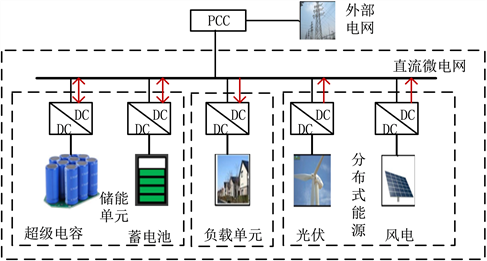Figure 1. Smart microgrid composition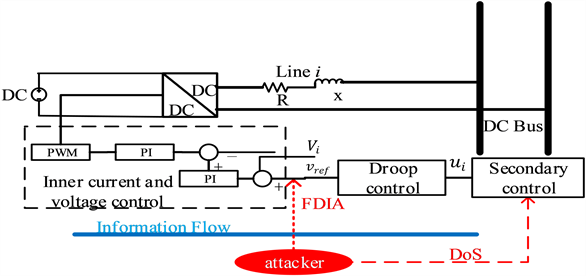Figure 2. Types of network attacks

2.1. 拒绝服务攻击

DoS攻击会造成系统资源不能用和传输信息丢失。到目前为止，一些数学模型被用来定量分析网络攻击导致的系统性能下降，如排队模型、伯努利模型或马尔可夫模型。本文考虑了一种通用的DoS攻击模型，该模型利用了DoS的两个典型特征，即DoS的频率和持续时间，而不需要对潜在的攻击策略提出任何要求，适用于各种DoS攻击 。为了定量描述DoS攻击的影响，让 $\left({d}_{n},{d}_{n}+{g}_{n}\right)\triangleq {D}_{n}\left({d}_{n}\ge 0,n\in N\right)$ 表示无攻击区间，此区间内干扰攻击信号停止且通信正常，且让 $\left({d}_{n}+{g}_{n},{d}_{n+1}\right)\triangleq {G}_{n}\left({d}_{n}+{g}_{n}<{d}_{n+1},n\in N\right)$ 在这个区间内，攻击信号活跃，信号不能被传输。为了便于描述，我们使用来 ${S}_{Dos}\left(t\right)$ 表示DoS攻击信号：

${S}_{Dos}\left(t\right)=\left\{\begin{array}{cc}0& \left({d}_{n},{d}_{n}+{g}_{n}\right)\\ 1& \left({d}_{n}+{g}_{n},{d}_{n+1}\right)\end{array}$ (1)

$n\left(t\right)\le \chi +\frac{t}{{\tau }_{v}}$ (2)

$|E\left(t\right)|\le \kappa +\frac{t}{\Gamma }$ (3)Figure 3. Event trigger control

2.2. 虚假数据注入攻击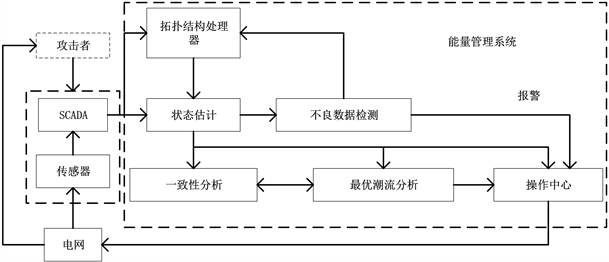Figure 4. Simplified operation diagram of smart microgrid

$z=\left[\begin{array}{c}{z}_{1}\\ {z}_{2}\\ ⋮\\ {z}_{m}\end{array}\right]=\left[\begin{array}{c}{h}_{1}\left({x}_{1}{x}_{2}{x}_{2n-1}\right)\\ {h}_{2}\left({x}_{1}{x}_{2}{x}_{2n-1}\right)\\ ⋮\\ {h}_{m}\left({x}_{1}{x}_{2}{x}_{2n-1}\right)\end{array}\right]+\left[\begin{array}{c}{e}_{1}\\ {e}_{2}\\ ⋮\\ {e}_{m}\end{array}\right]=h\left(x\right)+e$ (4)

$z=Hx+e$ (5)

H矩阵非零元素的数值是与各个支路上电抗的倒数有关，是一个雅可比矩阵，通过对交流非线性方程的 $h\left(x\right)$ 对各个状态变量求偏导数所得：

$H=\frac{\partial h\left(x\right)}{\partial x}=\left[\begin{array}{cccc}\frac{\partial {h}_{1}\left(x\right)}{\partial {x}_{1}}& \frac{\partial {h}_{1}\left(x\right)}{\partial {x}_{2}}& \cdots & \frac{\partial {h}_{1}\left(x\right)}{\partial {x}_{n-1}}\\ \frac{\partial {h}_{2}\left(x\right)}{\partial {x}_{1}}& \frac{\partial {h}_{2}\left(x\right)}{\partial {x}_{2}}& \cdots & \frac{\partial {h}_{2}\left(x\right)}{\partial {x}_{n-1}}\\ ⋮& ⋮& \ddots & ⋮\\ \frac{\partial {h}_{m}\left(x\right)}{\partial {x}_{1}}& \frac{\partial {h}_{m}\left(x\right)}{\partial {x}_{2}}& \cdots & \frac{\partial {h}_{m}\left(x\right)}{\partial {x}_{n-1}}\end{array}\right]$ (6)

H是一个 $m×\left(n-1\right)$ 维矩阵。针对上述量测方程模型(6)，将对系统进行状态估计，通过迭代逼近的方法使得方程组收敛，得到状态变量的估计值。加权最小二乘估计方法是电力系统状态估计中最常用的方法之一，它无需知道需要估计变量的统计特性，可以通过依据量测估计值 $H\stackrel{^}{x}$ 与量测值z之差的平方和最小的估计准则对系统未知变量进行估计。该方法假定量测误差e服从理想正态分布， $E\left({e}_{i}=0,i=1,\cdots ,m\right)$$E\left[{e}_{i},{e}_{j}\right]=0$，则最终估计结果具备最优一致且无偏的统计特性。基于此，定义量测残差为：

$y\left(x\right)=z-H\stackrel{^}{x}$ (7)

$\stackrel{^}{x}=\mathrm{arg}\mathrm{min}{\sum }_{i=1}^{m}W{\left({z}_{i}-Hx\right)}^{2}$ (8)

$\stackrel{^}{x}={\left({H}^{\text{T}}WH\right)}^{-1}{H}^{\text{T}}Wz$ (9)

$r=z-H\stackrel{^}{x}$ (10)

FDI攻击在信息层入侵智能电网，以 ${z}_{a}$ 表示被攻击后的系统量测值， $a={\left[\begin{array}{cccc}{a}_{1}& {a}_{2}& \cdots & {a}_{m}\end{array}\right]}^{\mathrm{T}}$ 表示数据注入的虚假数据向量。则实际的量测数据为 ${z}_{a}=z+a$ ；FDI引起状态变量的误差向量 $c={\left[\begin{array}{cccc}{c}_{1}& {c}_{2}& \cdots & {c}_{n}\end{array}\right]}^{\mathrm{T}}$，此时估计的状态变量为：

${\stackrel{^}{x}}_{a}=\stackrel{^}{x}+c$ (11)

$‖r‖=‖{z}_{a}-H{\stackrel{^}{x}}_{a}‖=‖z+a-H\left(\stackrel{^}{x}+c\right)‖=‖z-H\stackrel{^}{x}+a-Hc‖$ (12)

$‖r‖=‖{z}_{a}-H{\stackrel{^}{x}}_{a}‖=‖z-H\stackrel{^}{x}‖<\tau$ (13)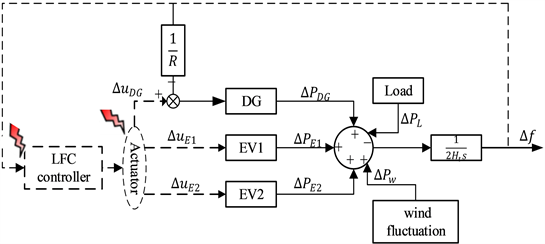Figure 5. Optimal elastic controller based on UIO and LQR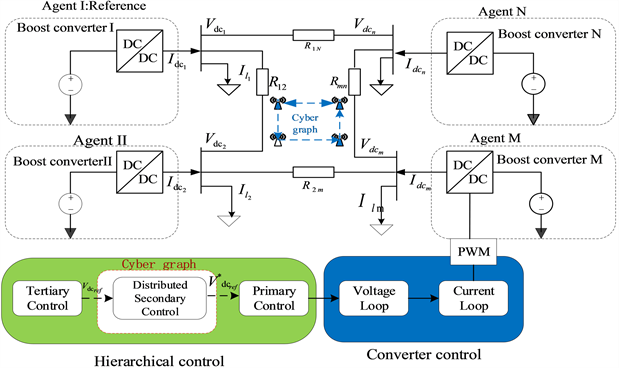Figure 6. CVF method detects hidden attacks

3. 网络攻击模式下的控制与检测策略分析

3.1. 基于多智能体一致性算法的协同控制

3.2. 基于智能微网的弹性分布式控制

3.3. 网络攻击检测

3.4. 其他控制方法

4. 总结与展望

  Afshari, A., Karrari, M., Baghaee, H.R., et al. (2020) Resilient Synchronization of Voltage/Frequency in AC Microgrids under Deception Attacks. IEEE Systems Journal, 15, 2125-2136. https://doi.org/10.1109/JSYST.2020.2992309  巴斯替, 骆德汉. 智能电网网络安全的发展与挑战[J]. 电工电气, 2019(7): 5-8+54.  Ding, L., Han, Q.L., Wang, L.Y., et al. (2018) Distributed Cooperative Optimal Control of DC Microgrids with Communication Delays. IEEE Transactions on Industrial Informatics, 14, 3924-3935. https://doi.org/10.1109/TII.2018.2799239  Liu, Y., Reiter, M.K. and Ning, P. (2009) False Data Injection Attacks Against State Estimation in Electric Power Grids. Proceedings of the 2009 ACM Conference on Computer and Communications Security (CCS 2009), Chicago, 9-13 November 2009, 21-32. https://doi.org/10.1145/1653662.1653666  Jiwei, T., Buhong, W., Fute, S., et al. (2017) Stealthy False Data Injection Attacks Using Matrix Recovery and Independent Component Analysis in Smart Grid. IOP Conference Series: Materials Science and Engineering, 199, Article ID: 012034. https://doi.org/10.1088/1757-899X/199/1/012034  Che, L., Liu, X., Li, Z., et al. (2019) False Data Injection Attacks Induced Sequential Outages in Power Systems. IEEE Transactions on Power Systems, 34, 1513-1523. https://doi.org/10.1109/TPWRS.2018.2871345  罗小元, 朱鸣皋, 王新宇, 关新平. 基于自适应卡尔曼滤波器的智能电网隐蔽假数据攻击检测[J]. 信息与控制, 2018, 47(1): 16-21.  Srikantha, P. and Kundur, D. (2015) Denial of Service Attacks and Mitigation for Stability in Cyber-Enabled Power Grid. Innovative Smart Grid Technologies Conference, Washington DC, 18-20 February 2015, 1-5. https://doi.org/10.1109/ISGT.2015.7131827  Zhang, H., Cheng, P., Shi, L. and Chen, J.M. (2015) Optimal Denial-of-Service Attack Scheduling with Energy Constraint. IEEE Transactions on Automatic Control, 60, 3023-3028. https://doi.org/10.1109/TAC.2015.2409905  Liu, S., Liu, X.P. and Saddik, A.E. (2013) Denial-of-Service (DoS) Attacks on Load Frequency Control in Smart Grids. 2013 IEEE PES Innovative Smart Grid Technologies Conference, Washington DC, 24-27 February 2013, 1-6. https://doi.org/10.1109/ISGT.2013.6497846  郑凯中, 樊春霞. 基于事件触发的遥操作系统在DOS攻击下的安全控制[J]. 南京邮电大学学报: 自然科学版, 2021, 41(2): 77-84.  Persis, C.D. and Tesi, P. (2015) Input-to-State Stabilizing Control Under Denial-of-Service. IEEE Transactions on Automatic Control, 60, 2930-2944. https://doi.org/10.1109/TAC.2015.2416924  Hu, S., Yuan, P., Yue, D., et al. (2019) Attack-Resilient Event-Triggered Controller Design of DC Microgrids under DoS Attacks. IEEE Transactions on Circuits and Systems I: Regular Papers, 67, 699-710. https://doi.org/10.1109/TCSI.2019.2948015  王先培, 田猛, 董政呈, 朱国威, 龙嘉川, 代荡荡, 等. 输电网虚假数据攻击研究综述[J]. 电网技术, 2016, 40(11): 3406-3414.  田继伟,王布宏, 李腾耀, 尚福特, 曹堃锐. 智能电网虚假数据注入攻击研究进展与展望[J]. 信息安全与技术, 2019, 10(9): 73-84.  Khalghani, M.R., Solanki, J., Solanki, S.K., et al. (2020) Resilient Frequency Control Design for Microgrids under False Data Injection. IEEE Transactions on Industrial Electronics, 68, 2151-2162. https://doi.org/10.1109/TIE.2020.2975494  Sahoo, S., Mishra, S., Peng, C.H., et al. (2018) A Stealth Cyber Attack Detection Strategy for DC Microgrids. IEEE Transactions on Power Electronics, 34, 8162-8174. https://doi.org/10.1109/TPEL.2018.2879886  Ma, T., Zhang, Z. and Cui, B. (2022) Impulsive Consensus of Nonlinear Fuzzy Multi-Agent Systems under DoS Attack. Nonlinear Analysis: Hybrid Systems, 44, Article ID: 101155. https://doi.org/10.1016/j.nahs.2022.101155  Li, S., Zou, W.C., Guo, J. and Xiang, Z.R. (2022) Consensus of Switched Nonlinear Multiagent Systems Subject to Cyber Attacks. IEEE Systems Journal. https://doi.org/10.1109/JSYST.2021.3110501  Zhou, Q., Shahidehpour, M., Alabdulwahab, A. and Abusorrah, A. (2020) A Cyber-Attack Resilient Distributed Control Strategy in Islanded Microgrids. IEEE Transactions on Smart Grid, 11, 3690-3701. https://doi.org/10.1109/TSG.2020.2979160  张露元, 许寅, 吴翔宇, 王思家. 抵御虚假数据注入攻击的交流微电网分布式韧性控制[J/OL]. 电力系统自动化, 2022: 1-19. http://kns.cnki.net/kcms/detail/32.1180.TP.20220702.1235.004.html, 2022-07-13.  楼琦凯, 陈蓓, 丁勐, 牛玉刚. 虚假数据注入攻击下直流微电网的滑模控制[J/OL]. 控制与决策, 2022: 1-9. https://doi.org/10.13195/j.kzyjc.2021.0606, 2022-07-13.  黄玲, 孙晓宇, 蔺小娜, 郭婧. 具有DoS攻击非线性网络的动态事件触发控制[J/OL]. 控制理论与应用, 2022: 1-9. http://kns.cnki.net/kcms/detail/44.1240.TP.20210825.1312.012.html, 2022-07-13.  Xu, R., Rui, W., Guan, Z., et al. (2017) Achieving Efficient Detection against False Data Injection Attacks in Smart Grid. IEEE Access, 5, 13787-13798. https://doi.org/10.1109/ACCESS.2017.2728681  Yang, L., Zhang, X., Li, Z., et al. (2020) Detecting Bi-Level False Data Injection Attack Based on Time Series Analysis Method in Smart Grid. Computers & Security, 96, Article ID: 101899. https://doi.org/10.1016/j.cose.2020.101899  Zhang, H., Qi, Y., Wu, J., et al. (2018) DoS Attack Energy Management against Remote State Estimation. IEEE Transactions on Control of Network Systems, 5, 383-394. https://doi.org/10.1109/TCNS.2016.2614099  Xu, W., Ho, D., Zhong, J., et al. (2019) Event/Self-Triggered Control for Leader-Following Consensus over Unreliable Network with DoS Attacks. IEEE Transactions on Neural Networks and Learning Systems, 30, 3137-3149. https://doi.org/10.1109/TNNLS.2018.2890119  Liu, S., Hu, Z., Wang, X., et al. (2018) Stochastic Stability Analysis and Control of Secondary Frequency Regulation for Islanded Microgrids Under Random Denial of Service Attacks. IEEE Transactions on Industrial Informatics, 15, 4066-4075. https://doi.org/10.1109/TII.2018.2885170  Rong, F., Huang, X., Sun, J., et al. (2017) Stability Analysis of the Cyber Physical Microgrid System under the Intermittent DoS Attacks. Energies, 10, Article No. 680. https://doi.org/10.3390/en10050680